Courses

# Olympiad Test: Triangle - 2

## 10 Questions MCQ Test Mathematics Olympiad Class 7 | Olympiad Test: Triangle - 2

Description
This mock test of Olympiad Test: Triangle - 2 for Class 7 helps you for every Class 7 entrance exam. This contains 10 Multiple Choice Questions for Class 7 Olympiad Test: Triangle - 2 (mcq) to study with solutions a complete question bank. The solved questions answers in this Olympiad Test: Triangle - 2 quiz give you a good mix of easy questions and tough questions. Class 7 students definitely take this Olympiad Test: Triangle - 2 exercise for a better result in the exam. You can find other Olympiad Test: Triangle - 2 extra questions, long questions & short questions for Class 7 on EduRev as well by searching above.
QUESTION: 1

### In a ∆ABC, ∠A – ∠B = 33° and ∠B – ∠C = 18°, then ∠B = ?

Solution:

∠A = 33° + ∠B and ∠C = ∠B – 18°
∴ (33° + ∠B) + ∠B + (∠B – 18°) = 180°
⇒ 3∠B = 165° 17. (d) ⇒ ∠B = 55°

QUESTION: 2

### The sum of all angles of a triangle is

Solution:

The sum of all the angles of a triangle is 180°.

QUESTION: 3

### In the given figure, what value of x will make AOB a straight line?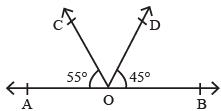Solution:
QUESTION: 4

In the given figure, AOB is a straight line,
∠AOC = (3x – 8)°, ∠COD = 50° and ∠BOD = (x + 10)°. The value of x is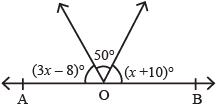Solution:

Here, (3x – 8)° + 50° + (x + 10)° = 180°
⇒ 4x + 52 = 180°
⇒ 4x = 180 – 52
⇒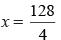⇒ x = 32

QUESTION: 5

In ∆ABC, ∠B = 90°, AB = 5cm and AC = 13cm then BC = ?

Solution:

By Pythagoras theorem,
AB2 + BC2 = AC2 ⇒ 52 + BC2 = 132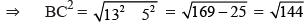= 12 cm

QUESTION: 6

∆ABC is an isosceles triangle with ∠C = 90° and AC = 5 cm then AB = ?

Solution:
QUESTION: 7

The angles of a triangle are (3x)°, (2x – 7)° and (4x – 11)°. Then x = ?

Solution:

According to question
3x + (2x – 7) + (4x – 11) = 180°
⇒ 9x – 18 = 180
⇒ 9x = 198
⇒ x = 22

QUESTION: 8

The supplement of 45° is

Solution:
QUESTION: 9

An angle is one – fifth of its supplement. The measure of the angle is

Solution:
QUESTION: 10

In the given figure, AOB is a straight line, ∠AOC = 68° and ∠BOC = x°, the value of x is.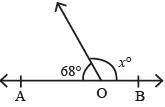Solution: